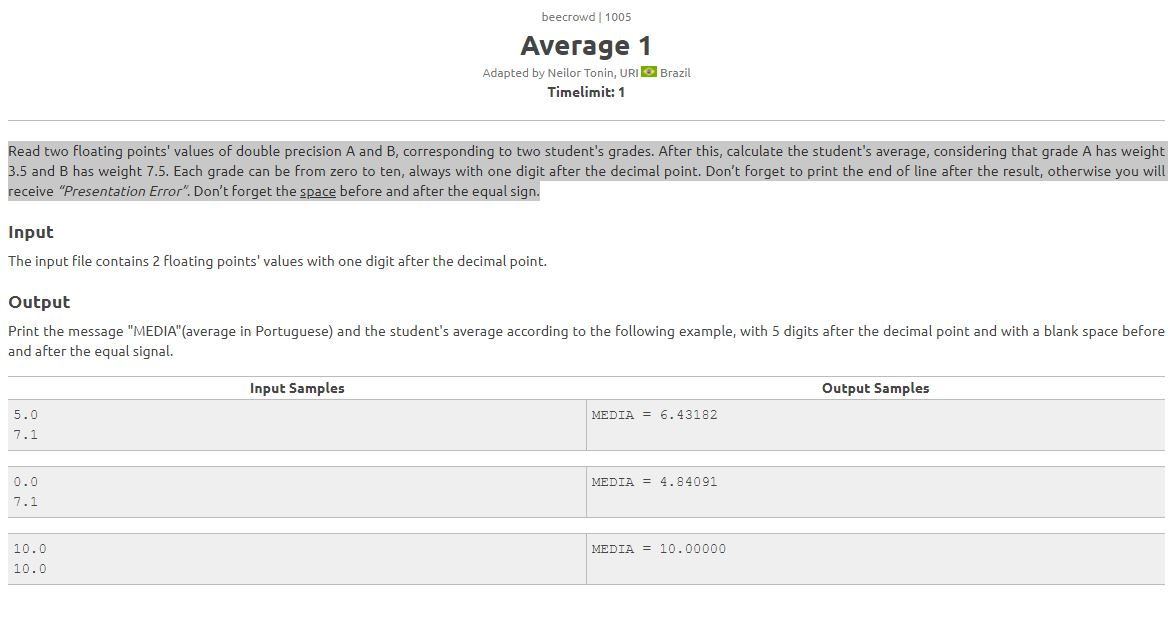# URI BEECROWD 1005 Average 1 Solution in C and Cpp and Python

URI BEECROWD 1005 Average 1 Solution in C and Cpp and Python

beecrowd | 1005
Average 1
Adapted by Neilor Tonin, URI  Brazil

Timelimit: 1
Read two floating points' values of double precision A and B, corresponding to two student's grades. After this, calculate the student's average, considering that grade A has weight 3.5 and B has weight 7.5. Each grade can be from zero to ten, always with one digit after the decimal point. Don’t forget to print the end of line after the result, otherwise you will receive “Presentation Error”. Don’t forget the space before and after the equal sign.

Input
The input file contains 2 floating points' values with one digit after the decimal point.

Output
Print the message "MEDIA"(average in Portuguese) and the student's average according to the following example, with 5 digits after the decimal point and with a blank space before and after the equal signal.

 Input Samples Output Samples 5.0 7.1 MEDIA = 6.43182 0.0 7.1 MEDIA = 10.00000 10.0 10.0 MEDIA = 10.00000

BEECROWD 1005 Average 1 Solution in C

``````

#include <stdio.h>
int main()
{
float A, B, MEDIA;
scanf("%f %f", &A, &B);
A= A*3.5;
B=B*7.5;
MEDIA = (A+B) /(3.5+7.5);
printf("MEDIA = %.5f
", MEDIA);
return 0;
}
```
```

BEECROWD 1005 Average 1 Solution in C++

``````

#include <bits/stdc++.h>

using namespace std;

int main() {

float A, B, MEDIA ;
cin>>A;
cin>>B;

A= A*3.5;
B=B*7.5;
MEDIA = (A+B) /(3.5+7.5);
cout<< fixed <<setprecision(5)<<"MEDIA = "<<MEDIA<<endl;
return 0;
}
```
```

BEECROWD 1005 Average 1 Solution in Python

``````
A= float(input())

B= float(input())

A=A*3.5

B=B*7.5

MEDIA = (A+B) / (3.5+7.5)

print("MEDIA = {:.5f}".format(MEDIA))
```
```

Explain BEECROWD 1005 Average 1:
• #include <bits/stdc++.h>: This line includes a common header file that includes most of the standard C++ library headers. It's a convenient way to include all the standard libraries in one line.
• using namespace std;: This line allows you to use standard C++ classes and functions without explicitly qualifying them with the std:: namespace.
• int main() { ... }: This is the main function where your program execution starts.
• float A, B, MEDIA;: This declares three floating-point variables A, B, and MEDIA to store the input grades and the calculated average.
• cin >> A;: This line reads the first floating-point value from the standard input and stores it in the variable A.
• cin >> B;: This line reads the second floating-point value from the standard input and stores it in the variable B.
• A = A * 3.5;: Multiplies the value of A by its weight, which is 3.5.
• B = B * 7.5;: Multiplies the value of B by its weight, which is 7.5.
• MEDIA = (A + B) / (3.5 + 7.5);: Calculates the weighted average by adding the weighted values of A and B and dividing by the sum of the weights.
• cout << fixed << setprecision(5) << "MEDIA = " << MEDIA << endl;: This line prints the result. fixed ensures that the output is shown with a fixed number of decimal places, and setprecision(5) sets the precision of the output to 5 decimal places. The calculated average MEDIA is printed after "MEDIA = ", and endl is used to print a newline character at the end.
• return 0;: This line indicates the end of the main function and returns an exit status of 0 to the operating system, indicating successful execution.

beecrowd-solution, uri online judge, beecrowd 1005 solution, beecrowd Average 1, beecrowd 1005, beecrowd python, beecrowd 1005 solution, beecrowd 1005 in cpp, beecrowd 1005 in c++, beecrowd 1005 in python, beecrowd Average 1 Solution, beecrowd 1005 solution pdf, beecrowd 1005 solution in c, beecrowd 1005 solution in python, beecrowd solution, BEE 1005, URI Problem 1005 Solution in C, URI Problem 1005 Solution in C++, BEECROWD Problem 1005 Solution in C, BEECROWD Problem 1005 Solution in C++